# UncertainLMH distribution

(Redirected from CumUncertainLMHInv)

New in Analytica 5.0

## UncertainLMH( xLow, xMedian, xHigh, pLow, lb, ub)

A simple and convenient way to specify a smooth probability distribution with three points, «xLow», «xMedian» and «xHigh». By default it assumes these parameters are the 10th, 50th, and 90th percentiles. Or you can specify the optional «pLow» probability percentile for «xLow», and it uses 1- «pLow» for «xHigh». For example,

UncertainLMH(10, 20, 30, pLow: 25%)

treats the first three parameters as the 25th, 50th, and 75th percentiles. «xMedian» is always the median.

You can set a lower bound, upper bound, or both: The distribution is unbounded below unless you specify a value for «lb» (lower bound). Similarly it is unbounded above unless you specify a value for «ub» (upper bound).

## Examples

An unbounded smooth continuous distribution with a 10% probability of being <= 8, a 50% probability of being <= 29, and a 90% probability of being less that 44. In other words, the 10-50-90 estimates are 8,29,44:

UncertainLMH(8, 29, 44)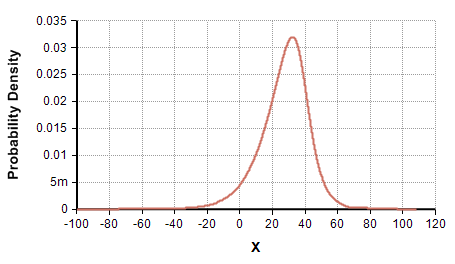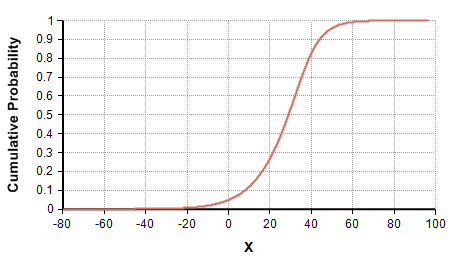A positive-only (semi-bounded) distribution with 10-50-90 percentiles of 8, 29 and 44:

UncertainLMH(8, 29, 44, lb:0)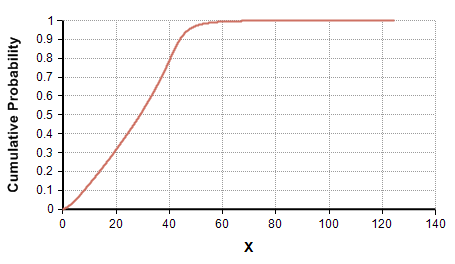A semi-bounded from above distribution with 10-50-90 percentiles of 8, 29, 44 and an upper bound of 60

UncertainLMH(8, 29, 44, ub:60)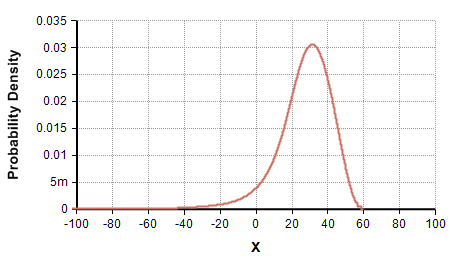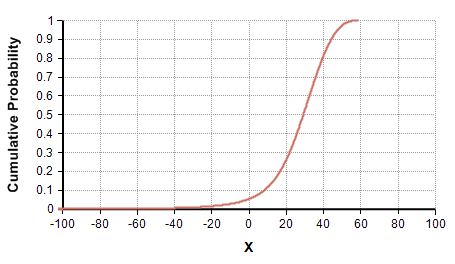A full bounded (between 0 and 60) distribution with 10-50-90 percentiles of 8, 29 and 44.

UncertainLMH(8, 29, 44, lb:0 ub:60)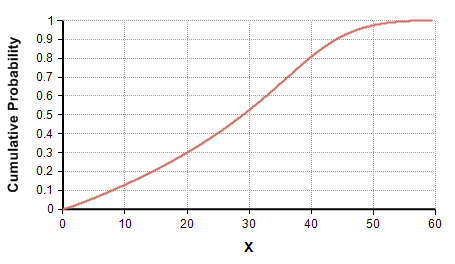Comparison of three unbounded distributions with different percentile levels. 5-50-95, 10-50-90 and 25-50-75. Notice that the CDF's value at X=8 is equal to the percentile level, that all three CDF curves pass through (29, 0.5), and that the CDF at x=44 is 1 minus the percentile level.

Index percentile := [5%, 10%, 25%] Do UncertainLMH(8, 29, 44, percentile)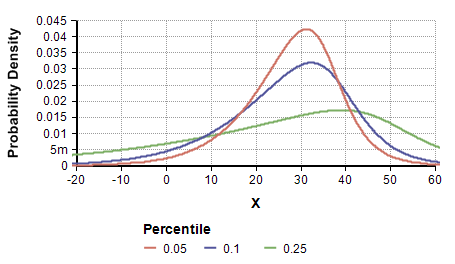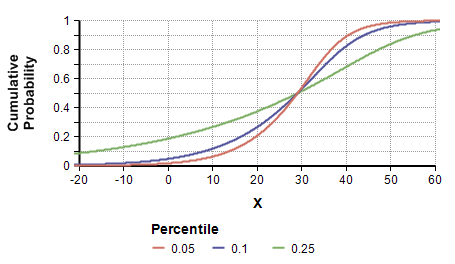(Note: Currently these graphs are from the Keelin SPT («method»=2) method. Results with the HBJ-QPD versions are quite similar).

## Analytic distribution functions

These analytic distribution functions compute the exact metric at a point on a distribution with no sampling error:

### DensUncertainLMH( x, xLow, xMedian, xHigh, pLow, lb, ub)

The probability density function. Returns the probability density at «x».

### CumUncertainLMH( x, xLow, xMedian, xHigh, pLow, lb, ub)

The cumulative probability function, computes the probability that the true value (or a value sampled from the distribution) is less than or equal to «x».

### CumUncertainLMHInv( p, xLow, xMedian, xHigh, pLow, lb, ub)

The inverse cumulative probability function, also called the quantile function. Returns the value x where there is a «p» probability of the true value (or a value sampled randomly from the distribution) being less than or equal to x.

## Method

By default, the method it uses to fit a smooth distribution to the parameters is a Hadlock-Bickel-Johnson Quantile-Parameterized Distribution, from:

• Christopher C. Hadlock and J. Eric Bickel (2017), "Johnson Quantile-Parameterized Distributions", Decision Analysis 14(1): 35-64.

Or if you set «method» parameter to 2 it uses a sample from a 3-term Keelin distribution with a Symmetric Percentile Triplet (SPT), a special case of the full metalog or Keelin distribution, also available in Analytica:

You can also set the the system variable, UncertainLMH_Method to 2, to get the Keelin SPT algorithm by default for the UncertainLMH distribution.

We recommend sticking with the default HBJ-QPD algorithm, because the Keelin/Metalog is subject to infeasible parameter combinations (see #Feasibility), unless you have particular reasons to prefer the Keelin SPT algorithm.

See #Analytic distribution functions below for functions that give exact values for the cumulative, inverse cumulative, and density functions.

## Feasibility

The metalog or Keelin SPT distribution finds some combinations of parameters infeasible. (The HBJ-QPB algorithm default since Analytica 5.4 does not suffer from these problems.) This section applies only if you have explicitly selected «method»=2.

The parameters must be ordered as «lb» < «xLow» < «xMedian» < «xHigh» < «ub». But, not all ordered parameters result in a valid 3-term Keelin distribution. Those combinations that are valid are called feasible, and parameter combinations that cannot be fit exactly are called infeasible. Infeasible combinations are typically very extreme. If the parameters are infeasible, an error message identifies the range of possible values for «xMedian» that would be feasible given the other parameters.

For the unbounded case, the parameter combination is feasible when (Keelin 2016, Proposition 2)

$\displaystyle{ k \lt r \lt 1-k }$

where

$\displaystyle{ k = {1\over 2} ( 1 - 1.66711 ({1\over 2} - p_{low} ) }$
$\displaystyle{ r = {{x_{median} - x_{low}} \over { x_{high} - x_{low} } } }$
k = 0.16658 in the 10-50-90 case.

## History

We introduced UncertainLMH() function in Analytica 5.0 using the SPT Keelin distribution. In Analytica 5.4, we switched it to use the Hadlock-Bickel-Johnson Quantile-Parameterized distribution by default to avoid issues with infeasible parameters.